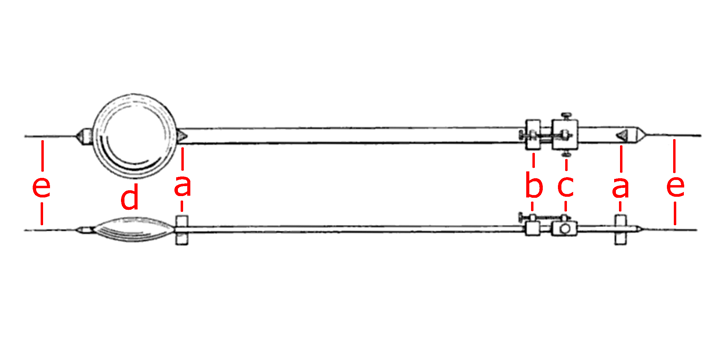# If It Ain’t Got That Swing

In History by Brian Koberlein1 Comment

A pendulum is a remarkably simple device that can be used in a range of scientific experiments. It can be used to measure the Earth’s rotation, for example. It can also be used as a timing mechanism. The period at which a pendulum swings depends upon the distribution of mass throughout the pendulum (known as the moment of inertia) and the distance of the swing point from its balance point (center of mass). The period also depends upon the acceleration of gravity, known as g. Because of that you can also use a pendulum to measure Earth’s gravity.

Nowadays, measuring g with a pendulum is a common lab in introductory physics. It can be done with a simple pendulum and a stopwatch. It can be a nice little exercise, but it isn’t overly accurate and requires a (now common) precision clock. But imagine trying to do such an experiment in the 1800s.  At that time pendulum clocks were the standard for measuring time, and they were calibrated to the stars. Pendulum clocks can be quite precise, but using one to measure g wasn’t particularly effective.

In 1817, Henry Kater developed a new kind of pendulum that could be used to determine g. It is now known as a Kater pendulum, and is a remarkably elegant device. A Kater pendulum relies upon the fact that for any pendulum there are two points where the period of oscillation is the same. If you pivot an object about a point and measure its period, there is a point on the other side of the object where period is the same.  Once those two points were determined, the value of g could be determined by the distance between the points and the period of oscillation, both of which could be measured with precision.

What made Kater’s pendulum so effective was that instead of taking an object and finding the points, he fixed the points and adjusted the distribution of mass to make them equal. In this way Kater could adjust mass until the periods were the same, then simply measure the period to determine g.  The measurement isn’t trivial, but it can be done to great accuracy.

When Kater performed this experiment, he got a value for g of 9.81158 meters per second squared, which is only a twentieth of a percent different from the modern value. The result was so precise, that it was used to determine the length of a “seconds pendulum”, which is mass connected to a length of wire so that its period is precisely 2 seconds (or each swing marks a second).

Interestingly, this length was proposed as a definition for the length of a meter. The official meter was later defined by the circumference of the Earth, but a modern meter is close to the length of a seconds pendulum. For this reason, if you take the square of pi it is almost equal to g. Just why that’s the case is something I’ll leave as an exercise for the reader.

1.